International
Tables for
Crystallography
Volume B
Reciprocal space
Edited by U. Shmueli

International Tables for Crystallography (2010). Vol. B, ch. 3.4, pp. 453-454   | 1 | 2 |

## Section 3.4.6. The case of n = 1 (Coulombic lattice energy)

D. E. Williamsa

aDepartment of Chemistry, University of Louisville, Louisville, Kentucky 40292, USA

### 3.4.6. The case of n = 1 (Coulombic lattice energy)

| top | pdf |

As taken above, the limit of the reciprocal-latticeterm oforexisted only if n was greater than 3. The corresponding contributions towere terms (5) and (9) of Section 3.4.5. To extend the method towe will show in this section that theseterms vanish if conditions of unit-cell neutrality and zero dipole moment are satisfied.

The integral representation of the term (5) is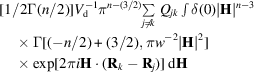and for term (9) is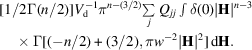Combining these two sums of integrals into one integral sum gives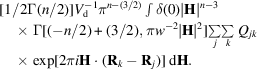For, supposeare net atomic charges so that the geometric combining law holds for. Then the double sum over j and k can be factored so that the limit that needs to be considered isIf the unit cell does not have a net charge, the sum over the q's goes to zero in the limit and this is a 0/0 indeterminate form. Letapproach zero along the polar axis so that, where subscript 3 indicates components along the polar axis. To find the limit with L'Hospital's rule the numerator and denominator are differentiated twice with respect to. Represent the numerator of the limit by the productand note that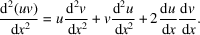It is seen that in addition to cell neutrality the product of the first derivatives of the sums must exist. These sums areandwhich vanish if the unit cell has no dipole moment in the polar direction, that is, if. Since the second derivative of the denominator is a constant, the desired limit is zero under the specified conditions. Now the polar direction can be chosen arbitrarily, so the unit cell must not have a dipole moment in any direction for the limit of the numerator to be zero. Thus we have the formula for the Coulombic lattice sum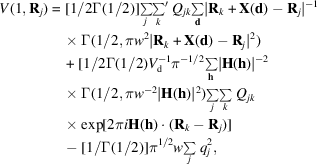which holds on conditions that the unit cell be electrically neutral and have no dipole moment. If the unit cell has a dipole moment, the limiting value discussed above depends on the direction of H. For methods of obtaining the Coulombic lattice sum where the unit cell does have a dipole moment, the reader is referred to the literature (DeWette & Schacher, 1964; Cummins et al., 1976; Bertaut, 1978; Massidda, 1978).

### References

Bertaut, E. F. (1978). The equivalent charge concept and its application to the electrostatic energy of charges and multipoles. J. Phys. (Paris), 39, 1331–1348.
Cummins, P. G., Dunmur, D. A., Munn, R. W. & Newham, R. J. (1976). Applications of the Ewald method. I. Calculation of multipole lattice sums. Acta Cryst. A32, 847–853.
DeWette, F. W. & Schacher, G. E. (1964). Internal field in general dipole lattices. Phys. Rev. 137, A78–A91.
Massidda, V. (1978). Electrostatic energy in ionic crystals by the planewise summation method. Physica (Utrecht), 95B, 317–334.